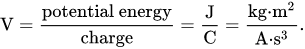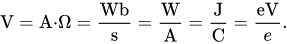Megavolts (MV) to volts (V) free online conversion

Megavolts (MV) to volts (V) conversion - calculator and how to convert.

Megavolts to volts conversion calculator

Enter the voltage in megavolts and press the Convert button:

MV
V

Volts to MV free online conversion »

How to convet megavolts to volts

1 MV = 106 V = 1000000 V

or

1 V = 10-6 MV = 0.000001 MV

Megavolts to volts formula

The voltage V in megavolts (MV) is equal to the voltage V in volts (V) times 1000000:

V(V) = V(MV) × 1000000

Example

Convert 3 megavolts to volts:

V(V) = 3MV × 1000000 = 3000000 V

Megavolts to volts conversion table

Megavolts (MV) Volts (V)
0 MV 0 V
0.000001 MV 1 V
0.00001 MV 10 V
0.0001 MV 100 V
0.001 MV 1000 V
0.01 MV 10000 V
0.1 MV 100000 V
1 MV 1000000 V

Volt definition

One volt is defined as the electric potential between two points of a conducting wire when an electric current of one ampere dissipates one watt of power between those points. Equivalently, it is the potential difference between two points that will impart one joule of energy per coulomb of charge that passes through it. It can be expressed in terms of SI base units (m, kg, s, and A) as:It can also be expressed as amperes times ohms (current times resistance, Ohm's law), webers per second (magnetic flux per time), watts per ampere (power per unit current, definition of electric power), or joules per coulomb (energy per unit charge), which is also equivalent to electronvolts per elementary charge:One Volt is defined as energy consumption of one joule per electric charge of one coulomb.

1V = 1J/C

One volt is equal to current of 1 amp times resistance of 1 ohm:

1V = 1A ⋅ 1Ω

Currently, we have around 1977 calculators, conversion tables and usefull online tools and software features for students, teaching and teachers, designers and simply for everyone.